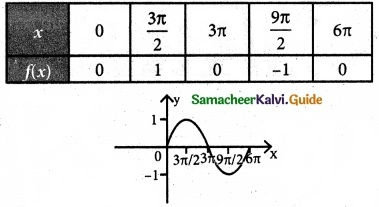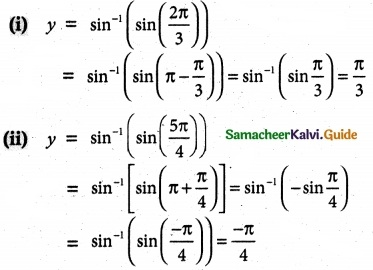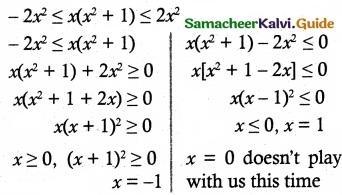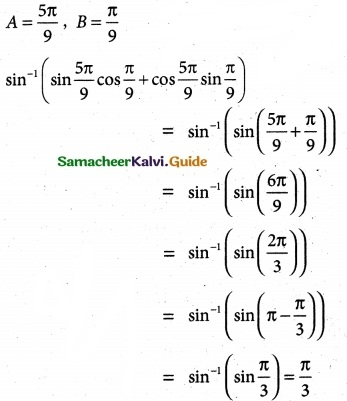Tamilnadu State Board New Syllabus Samacheer Kalvi 12th Maths Guide Pdf Chapter 4 Inverse Trigonometric Functions Ex 4.1 Textbook Questions and Answers, Notes.

## Tamilnadu Samacheer Kalvi 12th Maths Solutions Chapter 4 Inverse Trigonometric Functions Ex 4.1

Question 1.
Find all the values of x such that
Solution:
(i) sin x = 0
⇒ x = nπ
where n = 0, ±1, ±2, ±3, ……., ±10
(ii) sin x = -1
⇒ x = (4n – 1) $$\frac{\pi}{2}$$, n = 0, ±1, ±2, ±3, 4

Question 2.
Find the period and amplitude of
Solution:
(i) y = sin 7x
For Amplitude use the form
y = a sin(bx – c) + d
Amplitude = |a|
a = 1, ∴ |a| = 1
Period using the formula = $$\frac{2π}{|b|}$$ = $$\frac{2π}{|7|}$$ = $$\frac{2π}{7}$$
∴ Amplitude = 1
Period = $$\frac{2π}{7}$$

(ii) y = -sin ($$\frac{1}{3}$$x)
a = -1, b = $$\frac{1}{3}$$
∴ Amplitude = |a| = |-1| = 1
Period = $$\frac{2π}{|b|}$$ = $$\frac{2π}{| \frac{1}{3}|}$$ = 3(2π) = 6π

(iii) y =4 sin (-2x)
a = 4, b = -2
Amplitude = |4| = 4
Period = $$\frac{2π}{|b|}$$ = $$\frac{2π}{|-2|}$$ = $$\frac{2π}{2}$$ = πQuestion 3.
Sketch the graph of y = sin ($$\frac{1}{3}$$x) for 0 ≤ x ≤ 6π
Solution:Question 4.
Find the value of
(i) sin-1 (sin($$\frac{2π}{3}$$))
(ii) sin-1 (sin($$\frac{5π}{4}$$))
Solution:Question 5.
For what value of x does sin x = sin-1 x?
Solution:
sin x = sin-1 x is possible only when x = 0 (∵ x ∈ R)

Question 6.
Find the domain of the following
(i) f(x) = sin-1 ($$\frac{x²+1}{2x}$$)
Solution:
f(x) = sin-1 ($$\frac{x²+1}{2x}$$)
Range of sin-1x is [-1, 1]
-1 ≤ $$\frac{x²+1}{2x}$$ ≤ 1
Multiply by 2x² ≥ 0∴ solution is [-1, 1](ii) g (x) = 2 sin-1 (2x – 1) – $$\frac{π}{4}$$
Range of 2 sin-1 x is [-1, 1]
-1 ≤ 2x – 1 ≤ 1
2x – 1 ≤ 1 (or) 2x – 1 ≥ -1
2x ≤ 2 (or) 2x ≥ -1 + 1
x ≤ 1 (or) 2x ≥ 0 ⇒ x ≥ 0
x ≥ 0 and x ≤ 1
∴ solution is [0, 1]

Question 7.
Find the value of
(sin$$\frac{5π}{9}$$ cos$$\frac{π}{9}$$ + cos$$\frac{5π}{9}$$ sin$$\frac{π}{9}$$)
Solution:
sin (A + B) = sin A cos B + cos A sin B